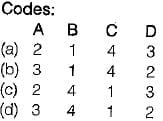Test: Basic Concept - 2

# Test: Basic Concept - 2

Test Description

## 10 Questions MCQ Test Thermodynamics | Test: Basic Concept - 2

Test: Basic Concept - 2 for Mechanical Engineering 2023 is part of Thermodynamics preparation. The Test: Basic Concept - 2 questions and answers have been prepared according to the Mechanical Engineering exam syllabus.The Test: Basic Concept - 2 MCQs are made for Mechanical Engineering 2023 Exam. Find important definitions, questions, notes, meanings, examples, exercises, MCQs and online tests for Test: Basic Concept - 2 below.
Solutions of Test: Basic Concept - 2 questions in English are available as part of our Thermodynamics for Mechanical Engineering & Test: Basic Concept - 2 solutions in Hindi for Thermodynamics course. Download more important topics, notes, lectures and mock test series for Mechanical Engineering Exam by signing up for free. Attempt Test: Basic Concept - 2 | 10 questions in 30 minutes | Mock test for Mechanical Engineering preparation | Free important questions MCQ to study Thermodynamics for Mechanical Engineering Exam | Download free PDF with solutions
 1 Crore+ students have signed up on EduRev. Have you?
Test: Basic Concept - 2 - Question 1

### Which of the following aspect is not true regarding microscopic propertie s of thermodynamic system

Test: Basic Concept - 2 - Question 2

### Choose the correct statement among the following:

Test: Basic Concept - 2 - Question 3

### Match List-I with List-I I and select the correct answer: List-I A. Interchange of matter is not possible in a B. Any processes in which the system returns to its original condition or state is called C. Interchange of matter is possible in a D. The quantity of matter under consideration in thermodynamics is called List-ll 1. Open system 2. System 3. Closed system 4. CycleTest: Basic Concept - 2 - Question 4

Which one of the following represents open thermodynamic system?

Test: Basic Concept - 2 - Question 5

A thermodynamic system is considered to be an isolated one if

Test: Basic Concept - 2 - Question 6

A control volume is

Test: Basic Concept - 2 - Question 7

Athermodynamic system refers to

Detailed Solution for Test: Basic Concept - 2 - Question 7

Explanation : A thermodynamic system is a quantity of matter of fixed identity, around which we can draw a boundary The boundaries may be fixed or moveable. Work or heat can be transferred across the system boundary. Everything outside the boundary is the surroundings.

When working with devices such as engines it is often useful to define the system to be an identifiable volume with flow in and out. This is termed a control volume.

A closed system is a special class of system with boundaries that matter cannot cross. Hence the principle of the conservation of mass is automatically satisfied whenever we employ a closed system analysis. This type of system is sometimes termed a control mass.

Test: Basic Concept - 2 - Question 8

In highly rarefied gases, the concept of this loses validity

Detailed Solution for Test: Basic Concept - 2 - Question 8

The concept of continuum loses validity when the mean free path of the molecules approaches the order of magnitude of the dimension of the vessel. So, in highly rarefied gases the concept of continuum loses its validity.

Test: Basic Concept - 2 - Question 9

Which of the following is an example of heterogeneous system?

Test: Basic Concept - 2 - Question 10

Consider the following:

1. Entropy
2. Viscosity
3. Temperature
4. Specific heat at constant volume

Which of the above properties of a system is/are intensive

Detailed Solution for Test: Basic Concept - 2 - Question 10

Concept:

Properties

All measurable characteristics of a system are known as properties.

Eg. Pressure, volume, temperature, etc.

There are two types of properties:

Extensive property

• Those properties which depend on mass are known as extensive properties.
• Examples are volume, energy, enthalpy, entropy etc.

Intensive property

• Those properties which don't depend on mass are known as intensive properties.
• Examples are pressure, temperature, density, viscosity.

## Thermodynamics

33 videos|54 docs|36 tests
Information about Test: Basic Concept - 2 Page
In this test you can find the Exam questions for Test: Basic Concept - 2 solved & explained in the simplest way possible. Besides giving Questions and answers for Test: Basic Concept - 2, EduRev gives you an ample number of Online tests for practice

## Thermodynamics

33 videos|54 docs|36 tests(Scan QR code)